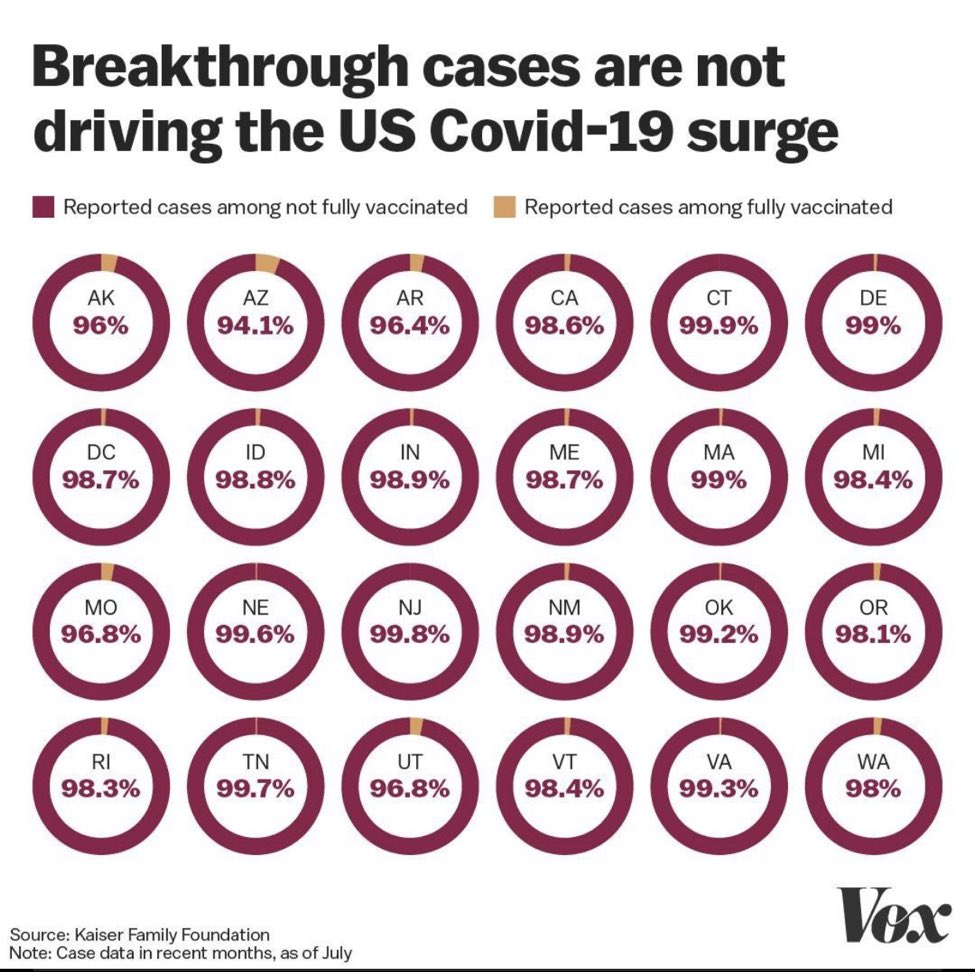# Some Covid Donuts To End The Week

Vox grabbed some data from the Kaiser Family Foundation and did a story a few days ago on it, then posted a different visualization of it that attracted some attention:I’m a pretty ardent donut detractor, but I have to also admit that they work pretty well for this use case, and we can reproduce the graphic in R quite nicely. The following code chunk:

• scrapes the datawrapper chart Vox embedded in their original article
• extracts the data from it using V8
• reformats it and uses some basic ggplot2 idioms for making the facets
``````library(V8) # we need to execute javascript to get the data
library(ggtext) # pretty ggtext
library(stringi) # some basic string ops
library(rvest) # scraping!
library(hrbragg) # remotes::install_github("hrbrmstr/hrbragg")
library(tidyverse) # duh

ctx <- v8() # init the V8 engine

pg <- read_html("https://datawrapper.dwcdn.net/jlEL9/6/") # get the data — this is embedded in the Vox article

# clean up the javascript so we can evaluate it
html_nodes(pg, xpath = ".//script[contains(., 'DW_SVEL')]") %>%
html_text() %>%
stri_replace_first_fixed("window.", "") %>%
stri_replace_all_regex("window.__.*", "", multiline = TRUE) %>%
ctx\$eval()

# get the dat from the V8 engine and reshape it a bit, then join it with state abbreviations
ctx\$get("__DW_SVELTE_PROPS__")\$data %>%
col_names = c(
"state",
"Reported cases among fully vaccinated",
"Reported cases among not fully vaccinated"
)
) %>%
gather(measure, value, -state) %>%
left_join(
tibble(
state = state.name,
abbr = state.abb
) %>%
state = "District of Columbia",
abbr = "DC"
)
) -> voxxed

# basic ggplot idiom for faceted donuts

ggplot() +
geom_col(
data = voxxed,
aes(3, value, fill = measure), # play with "3" here and below to change width of the donut
color = NA,
position = position_stack()
) +
ggtext::geom_richtext(
data = voxxed %>% filter(measure == "Reported cases among not fully vaccinated"),
# change 0.2 and 0 to see what they do
aes(0.2, 0, label = sprintf("%s<br/><b><span style='color:#8a264a'>%s%%</span></b>", abbr, value)),
size = 5, label.size = 0
) +
scale_x_continuous(
limits = c(0.2, 3 + 0.5) # this 3 links to the 3 above. tweak 0.2 and 0.5 to see what it does to the shape
) +
scale_fill_manual(
name = NULL,
values = c(
"Reported cases among fully vaccinated" = "#d59e67",
"Reported cases among not fully vaccinated" = "#8a264a"
)
) +
coord_polar(theta = "y") +
facet_wrap(~state, ncol = 6) +
labs(
x = NULL, y = NULL,
title = "Breakthrough cases are not driving the US Covid-19 surge",
caption = "Source: Kaiser Family Foundation\nNote: Case data in recent months, as of July\nOriginal chart by Vox <https://www.vox.com/22602039/breakthrough-cases-covid-19-delta-variant-masks-vaccines>"
) +
theme_inter(grid="") +
theme(
axis.text.x = elb(),
axis.text.x.top = elb(),
axis.text.x.bottom = elb(),
axis.text.y = elb(),
axis.text.y.left = elb(),
axis.text.y.right = elb()
) +
theme(
panel.spacing.x = unit(0, "npc"),
panel.spacing.y = unit(0, "npc")
) +
theme(
strip.text = elb(),
strip.text.x = elb(),
strip.text.y = elb()
) +
theme(
legend.position = "top",
legend.text = elt(size = 12),
legend.justification = "left"
) +
theme(
plot.caption = elt(hjust = 0)
)
``````Amazon Author Page

## 4 Comments →Some Covid Donuts To End The Week

This site uses Akismet to reduce spam. Learn how your comment data is processed.